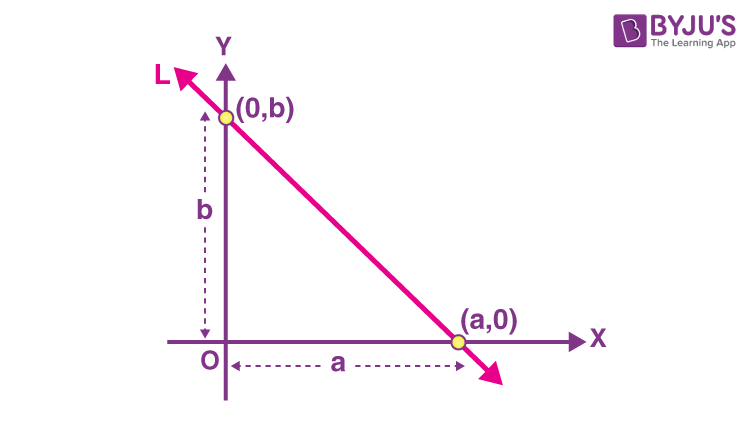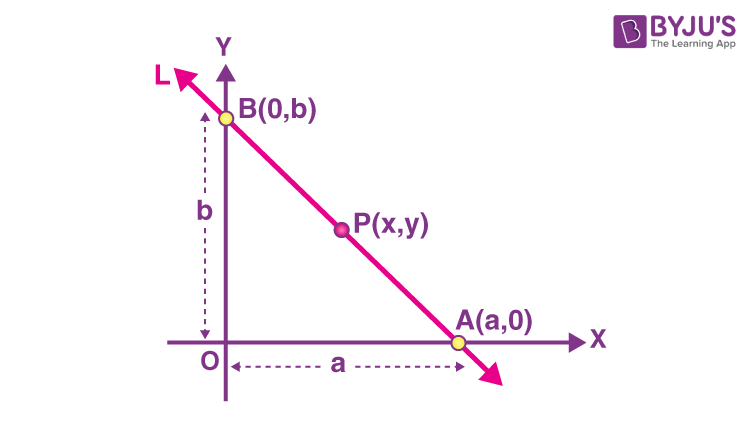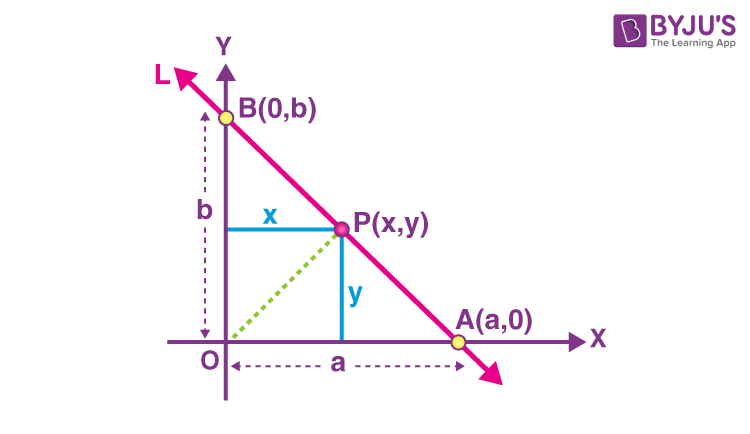# Intercept Form

In mathematics, we can write the equation of a given line in different ways based on the various parameters. The most commonly observed methods involve point-slope form, slope-intercept form, two-point form, intercept form, etc. All these methods deal with the deviation and expression of the equation of the given line using a point and slope, slope and y-intercept, two points on the line, intercepts of coordinates of the given points, etc., respectively. All these are explained in the chapter of straight lines class 11. In this article, you will learn the formula of the intercept form equation, how to derive the equation of straight line in intercept form using different methods.

## Intercept Formula

The equation for a straight line in intercept form is given by the formula,

$$\begin{array}{l}\large \frac{x}{a}+\frac{y}{b}=1\end{array}$$

Where,

a = x-intercept

b = y-intercept

This formula can also be derived using the general equation of a line.

## Intercept Form of a Straight Line

We can derive the equation of a straight line in intercept form in two ways. Let’s derive the formula of the intercept form of linear equation.

Method 1:

Suppose a line L makes x-intercept a and y-intercept b on the axes. That means L meets x-axis at the point (a, 0) and y-axis at the point (0, b), respectively as shown in the below figure.As we know, the equation of a line in two point form is:

$$\begin{array}{l}\large {y-y_{1}}=\frac{y_{2}-y_{1}}{x_{2}-x_{1}}(x-x_{1})\end{array}$$

Here,

(x1, y1) = (a, 0)

(x2, y2) = (0, b)

Substituting these coordinates in the above formula,

$$\begin{array}{l}y – 0=\frac{b-0}{0-a}(x-a)\end{array}$$

y = (-b/a) (x – a)

ay = -bx + ab

bx + ay = ab

Dividing by “ab” on both sides,

(bx/ab) + (ay/ab) = ab/ab

(x/a) + (y/b) = 1

Therefore, the equation of the line making intercepts a and b on x-and y-axis, respectively, is given as:

$$\begin{array}{l}\frac{x}{a}+\frac{y}{b}=1\end{array}$$

Method 2:

Consider the line L makes x-intercept a and y-intercept b on the axes such that L meets x-axis at the point (a, 0) and y-axis at the point (0, b), respectively, as shown in the above figure.

Thus, the x-intercept is “a” and the y-intercept is “b”.

Also, let (x1, y1) = (a, 0) and (x2, y2) = (0, b)

Slope of the line passing through the points (x1, y1) and (x2, y2) is:

m = (y2 – y1)/(x2 – x1)

So, the slope of line L is: m = (b – 0)/(a – 0) = -b/a

The equation of a straight line in slope-intercept form is:

y = mx + c

Here, m = slope and c = y-intercept

Substituting m = -b/a and y-intercept,

y = (-b/a)x + b

y = (-bx + ab)/a

ay = -bx + ab

bx + ay = ab

Dividing both sides of the equation by “ab”,

(bx/ab) + (ay/ab) = ab/ab

(x/a) + (y/b) = 1

Thus, this is the equation of line in intercept form.

Method 3:

Suppose the line L meets x-axis at the point A(a, 0) and y-axis at the point B(0, b), respectively. So, the x-intercept is a and y-intercept is b.

Let P(x, y) be the point on the line L such that the points A, P and B are collinear.We know that the area of the triangle formed by three collinear points is 0.

Thus, area of the triangle APB = 0

(½) |x1(y2 – y3) + x2(y3 – y1) + x3(y1 – y2)| = 0

Here,

(x1, y1) = (a, 0)

(x2, y2) = (x, y)

(x3, y3) = (0, b)

Substituting these values, we get;

(½) |a(y – b) + x(b – 0) + 0(0 – y)| = 0

ay – ab + bx + 0 = 0

bx + ay = ab

On dividing this equation by “ab” we get,

(bx/ab) + (ay/ab) = ab/ab

(x/a) + (y/b) = a

Hence, this is the required equation for the given line.

Method 4:

Suppose the line L meets x-axis at the point A(a, 0) and y-axis at the point B(0, b), respectively. So, the x-intercept is a and y-intercept is b.

Let P(x, y) be the point on the line L such that the points A, P and B are collinear. Then, join OP as shown in the below figure.Here, we can observe three triangles, namely ΔOAB, ΔOAP, and ΔOPB.

Also, ar(ΔOAB) = ar(ΔOAP) + ar(ΔOPB)

(1/2) × a × b = (1/2) × a × y + (1/2) × b × x {since area of triangle = (1/2) × base × height}

(ab/2) = (ay + bx)/2

ab = ay + bx

Dividing by “ab” on both sides,

ab/ab = (ay + bx)/ab

a = (ay/ab) + (bx/ab)

Therefore, (x/a) + (y/b) = 1.

### Intercept Form Examples

Example 1:

Find the equation of the line, which makes intercepts –4 and 5 on the x- and y-axes, respectively.

Solution:

Given,

x-intercept = a = -4

y-intercept = b = 5

Equation of the line in intercept form is:

(x/a) + (y/b) = 1

Substituting the values of a and b, we get the equation as:

(x/-4) + (y/5) = 1

Or

(-5x + 4y)/20 = 1

-5x + 4y = 20

5x – 4y + 20 = 0

Example 2:

Write the intercepts of the straight line represented by the equation 2x – 3y + 6 = 0 on the coordinate axes.

Solution:

Given line equation is:

2x – 3y + 6 = 0

2x – 3y = -6

Dividing both sides of the equation by -6,

(2x – 3y)/(-6) = (-6)/(-6)

(2x/-6) – (3y/-6) = 1

(x/-3) + (y/2) = 1

This is of the form (x/a) + (y/a) = 1.

So, a = -3 and b = 2

Therefore, the x-intercept is -3 and y-intercept is 2 for the given equation of a line.